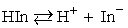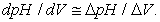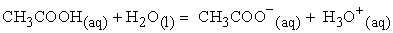﻿ Qualitative Measurement of pH and Mathematical Methods for the Determination of the Equivalence Point in Volumetric Analysis

### Qualitative Measurement of pH and Mathematical Methods for the Determination of the Equivalence Poin...

Andrea Checchetti, Jessica Lanzo

## Qualitative Measurement of pH and Mathematical Methods for the Determination of the Equivalence Point in Volumetric Analysis

Andrea Checchetti1,, Jessica Lanzo2,1Istituto d’Istruzione Superiore, “Leonardo da Vinci”, San Giovanni in Fiore, Italy

2Istituto Comprensivo, “Scopelliti-Green”, Rosarno, Italy

### Abstract

In this article is presented a gradual path on the pH making use of the laboratory. The idea arises from the consideration that the laboratory does not have to be the place of the ritual repetition of standardized procedures, often carried out with materials and instruments prepared, but a method of approach to phenomena and situations that promote the active participation of students. We move from a qualitative measure of the pH of acidic solutions, basic and neutral, to quantitative measure of the equivalent point of an acid-base titration. The methodology used by the teachers is based on learning by doing: few notions of theory to learn the fundamental concepts and many laboratory activities that help the students to produce reports on their activity. The qualitative measurement of pH, using as an indicator the red onion of Tropea (Italy), was carried out in a lower secondary school, while the volumetric analysis has been at the center of lab activity addressed to fourth class of a technical institute. In particular, the attention has been focused on acid-base titrations and how to determine the equivalence point. In both school levels, the primary objective was the acquisition of the fundamental principles and practical skills relating to the methods of analysis. All activities have been selected and organized in order to constitute a valid and mutual integration with the theory, not only as a moment of experimental verification of what the students have learned in theory, but also, where possible, as a basic tool to derive laws, principles theoretical models starting from the experience.

• Andrea Checchetti, Jessica Lanzo. Qualitative Measurement of pH and Mathematical Methods for the Determination of the Equivalence Point in Volumetric Analysis. World Journal of Chemical Education. Vol. 3, No. 3, 2015, pp 64-69. http://pubs.sciepub.com/wjce/3/3/2
• Checchetti, Andrea, and Jessica Lanzo. "Qualitative Measurement of pH and Mathematical Methods for the Determination of the Equivalence Point in Volumetric Analysis." World Journal of Chemical Education 3.3 (2015): 64-69.
• Checchetti, A. , & Lanzo, J. (2015). Qualitative Measurement of pH and Mathematical Methods for the Determination of the Equivalence Point in Volumetric Analysis. World Journal of Chemical Education, 3(3), 64-69.
• Checchetti, Andrea, and Jessica Lanzo. "Qualitative Measurement of pH and Mathematical Methods for the Determination of the Equivalence Point in Volumetric Analysis." World Journal of Chemical Education 3, no. 3 (2015): 64-69.

 Import into BibTeX Import into EndNote Import into RefMan Import into RefWorks

1234
Prev Next

### 1. Introduction

The path was born from the comparison of authors, currently attending a master on Teaching Science, sponsored by the Italian Ministry of Education. The authors both teach chemistry at two different levels respectively lower and higher secondary schools. The shared path offers various possibilities of articulation and development that it can be carried out in different contexts, considering the experiences and the knowledge of the students involved. The organization of this article reflects the observations and reflections of the students who produced reports, posters, charts, drawings, photographs and infographics. The methodological approach is similar for the two school levels. It differs only in the degree of formalization and the use of scientific language, which gradually becomes more specific when one moves from lower to higher secondary school.

### 2. Lower Secondary School

The reactions of acids and bases with water are measured using the pH scale. The students, when universal indicator is added to a solution, can clearly understand how the pH of a solution changes because the colour of the solution is changing. They can thus distinguish whether a solution is acidic, basic or neutral. They may, in the case of acid or basic solution, to understand the influence of the concentration of the dissolved substance in the water on the variation of pH by observing the chromatic variations of the solutions. Students learn, on the molecular level, that pH is a measure of the concentration of H3O+ ions in water and that adding an acid or a base to water affects the concentration of these ions.

2.1. Reagents and Materials

•  3 plastic cups

•  1 lemon (Citric acid) or commercial vinegar (acetic acid)

•  Sodium carbonate

•  Universal indicator solution

•  Water

Reagent for preparation of universal indicator solution

•  Onion of Tropea

•  1 Pot

•  1 Knife

•  Water

2.2. Experimental Part
2.2.1. Indicator

The pH scale runs from below 0 to 14. A solution with a pH of less than 7 is an acid, while one with a pH of greater than 7 is basic. A solution with a pH of 7 is neutral.

pH can be determined experimentally by using an indicator, a soluble dye. Its colour changes in short range of two units of the pH scale. Litmus and phenolphthalein are usually utilized: litmus often absorbed onto filter paper and phenolphthalein instead in acid-base titrations.

Red litmus changes from red to blue in the pH range from 6 to 8. Phenolphthalein changes from colorless to pink in the range from 8 to 10. Universal indicator papers, which contain a mixture of several indicators, change colour over a wide pH range (Figure 1).Download asVeiw figureFigures index
Figure 1. Litmus paper

They are usually weak acids or bases, but their conjugate base or acid forms have different colours due to differences in their absorption spectra.

Indicators are complicate organic weak acids or bases with complicated structures. For simplicity, we represent a general indicator by the formula HIn for acidic indicators. The ionization of acidic indicator in a solution is represented by the equilibrium :where "HIn" and "In" stand for the indicator molecule with and without an attached hydrogen ion.

Anthocyanins are a class of water-soluble pigments belonging to the family of flavonoids.

The color of anthocyanin can vary from red to blue-green depending on the pH of the medium in which they are located. This is also the case of the sauce of Tropea’s red onion. The red onion is rich in anthocyanins. Its liquid is colored purple, but in contact with acidic substances, it becomes red, while in contact with basic substances it becomes green. The colour shown by this indicator can be matched against a pH scale. The steps for its preparation are:

1. Cut the onion into slices and place in a pot

2. Add water to cover and boil for half an hour

2.2.2. Measure of pH

We used a solution of commercial vinegar and a solution of sodium bicarbonate. We measured the pH of solutions with red litmus paper and we obtained:

pH = 3 (red) for commercial vinegar,

pH = 12 (Blue) for sodium bicarbonate.

We repeated the same operation, this time adding a few drops of our indicator, the onion juice. The acid solution (Commercial Vinegar) in contact with the indicator gave a purple color, the basic solution (sodium bicarbonate), showed a green color, as shown in Figure 2 and Figure 3.Download asVeiw figureFigures index
Figure 2. Color of vinegar solution (Acid)Download asVeiw figureFigures index
Figure 3. Color of sodium carbonate solution (Base)Download asVeiw figureFigures index
Figure 4. pH meter

Measurements more precise can be obtained using an electronic pH meter (Figure 4). A pH meter determines pH from the electrical potential between two electrodes in a solution. A pH meter is used when reliable knowledge or control of pH is necessary.

In lower secondary school, we introduce the concept of acid-base reaction. In the laboratory in the reaction between a strong acid and a strong base, when combine stoichiometrically equivalent amount we call this stoichiometric mixture the equivalence point. In this point, there is a change in the color of our substance used as indicator. A simple method for finding the equivalence point is to control the mixture that changes color at pH of 7 (Figure 5).Download asVeiw figureFigures index
Figure 5. Change in the color of substance used as indicator

We demonstrated that the sauce of Tropea’s red onion is an good indicator for the qualitative determination of pH.

### 3. Higher Secondary School

In the titration of a strong acid with a strong base does not present particular problems, but in many cases we must resort to graphic methods or the calculation of the derived functions, first and second. The mathematical methods used in this article are based upon the assumption that for all titrations the equivalence point and the inflection point coincide. The mathematical methods include:

1. The method of the first derivative;

2. The method of the second derivative

3.1. Experimental Procedures

The activities of laboratory concerned:

1. Stoichiometry;

2. Preparation of solutions;

3. Behavior of acid-base indicators

4. Titrations of acidic and basic solutions

5. Determination of the titration curves

6. First derivative and second derivative calculated for performed titrations.

7. Determination of equivalent volume

3.2. Background

On the basis of the points of the titration curves (pH versus volume) you trace the first derivative calculated for points; this allows you to better identify the equivalence point of the titration, because the point of inflection of the first derivative of a function has a maximum or a minimum. Incremental ratios were calculated for a series of points close to the equivalence point. The incremental ratio expresses the average value of the slope of the titration curve in the interval between two points:The values of the incremental ratios are plotted on a graph from which we obtain the value of Ve at the point of maximum . In theory, the incremental ratios should be calculated on the basis of infinitesimal intervals, but in practice if the additions of titrant (V) are small enough we can assume thatIn the inflection point of the function, the second derivative vanishes and therefore the equivalence point of the titration corresponds to the second derivative of the function at the intersection with the x-axis.

3.3. Reagents and Materials

To perform titrations mentioned above from point 5 to point 7 it took

•  50 ml burette, precision ± 0.1 ml (to be filled with HCL and NaOH standard solutions);

•  HCL and NaOH standard solutions 0.1 M;

•  Phenolphthalein indicator;

•  Distillated water;

•  Multimeter to measure pH and mV;

•  Commercial vinegar;

•  Pepsi-cola.

### 4. Result and discussion

In Figure 6 is shown the titration curve of strong base-strong acid.Download asVeiw figureFigures index
Figure 6. Titration curve of sodium hydroxide-hydrochloric acidDownload asVeiw figureFigures index
Figure 7. Second derivative calculated for points starting from the first derivative for a titration of sodium hydroxide with hydrochloric acid

In Table 1 are reported the experimental values with which it was built the titration curve of Figure 6 and the trend of the second derivative function of Figure 7.

The Figure 2. shows the second derivative function around the inflection point. It can be asserted that the inflection point of the original function (as shown in Figure 1) is located between 11.3 and 11.35 mL.

#### Table 1. Experimental values of volume and pH together to incremental ratios for titration of sodium hydroxide-hydrochloric acidDownload asVeiw tableTables index

Figure 8 shows the titration curve of a commercial vinegar, while Figure 9 shows the graph of the function the first derivative versus mL of titrant NaOH. In Table 2 the related data to graph the curvesDownload asVeiw figureFigures index
Figure 8. The titration curve of a commercial vinegarDownload asVeiw figureFigures index
Figure 9. First derivative calculated for points for commercial vinegar and its inverse

#### Table 2. Experimental values of volume and pH together to incremental ratios for titration commercial vinegarDownload asVeiw tableTables index

In the same figure a better and easier intersection is obtained by plotting the inverse of the first derivative as interpolation in the neighborhood of equivalent point becomes linear, .

In the case of a weak acid, once determined the equivalent point, you can estimate the value of the equilibrium constant through the half-equivalence point. In fact, in the case of commercial vinegar the titration is used to determine the concentration of acetic acid. The reaction of equilibrium is the following:And Ka is equal toAt the half-titration point, exactly half of the acetic acid has been converted into its coniugate base and consequently [H3O+] = Ka or pH = pKa. In Table 2 the equivalent point occurs at 24,1 mL, the half-equivalent point at 12,05 mL and the pKa is approximatively 4,74 that is Ka = 1.812 × 10-5 in good agreement with the tabulated value, . The pH at half of that volume yields information about the dissociation constant. In the "half-way" region of the titration curve the data is frequently nearly linear and a least-squares fit of that portion of the data can aid in interpretation, .Download asVeiw figureFigures index
Figure 10. The titration curve of Pepsi-colaDownload asVeiw figureFigures index
Figure 11. Second derivative calculated for points starting from the first derivative for a titration of Pepsi-cola with sodium hydroxide

Finally, the last titration studied is related to the determination of phosphoric acid in pepsi-cola. In Figure 10 the titration curve, which shows two points of inflection. From Figure 11, it can be stated that the points, where the second derivative vanishes intersecting the x-axis, correspond to the equivalence points of the titration curve, respectively with Ve = 4.6 mL and Ve = 16.1 mL.

#### Table 3. Experimental values of volume and pH together to incremental ratios for titration pepsi-colaDownload asVeiw tableTables indexDownload asVeiw figureFigures index
Figure 12. Second derivative function in the neighborhood of inflection point

You can calculate the equivalence point by considering only the two points before and after which the second derivative vanishes (points A and C in Figure 12). The segment MN is given by the formula :Adding the value of MN to the value of the abscissa of the point M, you get the value of Ve. In Figure 11 are reported the values obtained for the two inflection points.

### 5. Conclusion

The laboratory activity has had the primary objective the acquisition of the fundamental principles and practical skills relating to the methods of qualitative and quantitative analysis with particular reference to the acidimetry.

We have experienced a gradual path of measurement of the pH moving from a qualitative measure to arrive at a quantitative measure all carried out through a teaching laboratory.

### Acknowledgement

This work was supported by the Istituto d’Istruzione Superiore “Leonardo da Vinci” of San Giovanni in Fiore (Italy) and the Istituto Comprensivo “Scopelliti-Green” of Rosarno (Italy).

### References

  Pathan Mohd Arif Khan et al, J Adv Scient Res, 2011, 2(4): 20-27.In article  Cozzi R., Protti P., Ruaro T., Analisi Chimica Strumentale, Vol. C, Bologna, Italy: Zanichelli Edition, 1997.In article  Università di Padova, Equilibri acido-Base. Available:http://www.chimica.unipd.it/ca1/AB_TUTTO_1.pdf.In article  Clark College, Potentiometric titration of a weak acid, 2005. Available:http://web.clark.edu/nfattaleh/classes/135/Sp05/Labs/PotTitrSp05.pdf.In article  Titration of a Diprotic Acid, Available:http://www.chemtopics.com/aplab/diprotic.pdf.In article